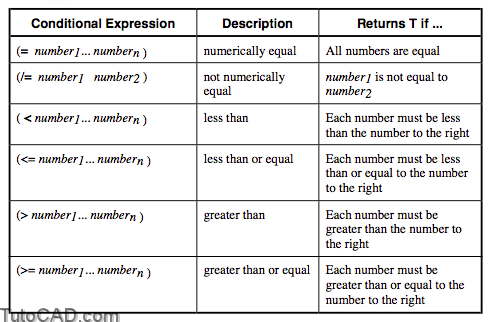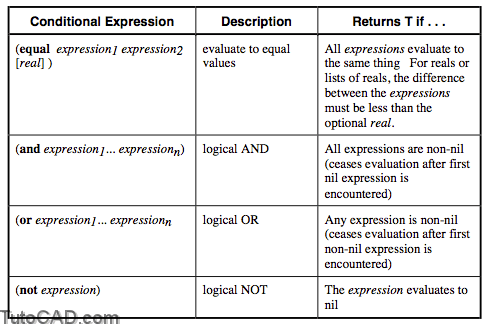Conditional Expressions with autolisp in AutoCAD

Sometimes you will want a program to do different things depending on different conditions. This is called branching. In order to create program branches, we need to construct “test conditions.” If the test is true, then we do one result; if false, a different result.

Conditional expressions can be used with several functions to achieve branching in AutoLISP routines.

1. Any expression can be used as a conditional.

In AutoLISP, any expression is true if it evaluates to a non-nil value. Any expression is false if it evaluates to nil. Hence, each of the following is a valid conditional expression:

(setq A 5)
PT1 – as long as it holds some value
(getpoint “Enter center point: “)

(setq A B)

2. Specific functions are designed to be conditional expressions.

These expressions return the value of “T” if true, and “nil” if false. They are divided into two types: Numerical (=,<,>, etc.) for numbers and Logical (equal to, less than, greater than, etc.) for other expressions.

Examples

Command: (= 5 5.0)
T

Command: (/= 5 (- 7 1))
T
Command: (setq G 3.0 H 2.96)
2.96
Command: (= G H)
nil
Command: (equal G H 0.1) – are G & H equal within 0.1?
T
Command: (equal G H 0.04) – are G & H equal within 0.04?
nil

Numerical Conditions

The following conditional expressions are valid for numbers and strings:Logical Conditions

The following conditionals will work with any expression:PRACTICE

Test the following expressions at the Command line.

(equal 0.1 0.2)
(equal 0.1 0.2 0.1)
(= “this” “that”)
(equal “this” “that”)
(or “this” nil “that”)
(and “this” nil “that”)
(> (+ 5(/ 4 5)) 8)